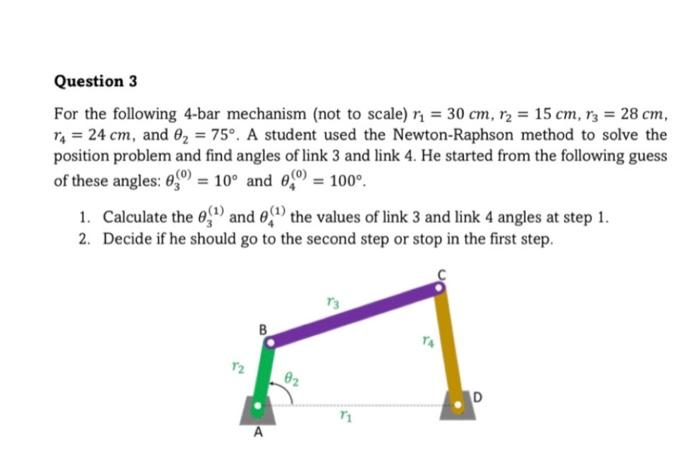Home / Expert Answers / Mechanical Engineering / i-hope-it-will-be-solved-by-handwritten-for-the-following-4-bar-mechanism-not-to-scale-r-1-3-pa727

# (Solved): I hope it will be solved by handwritten. For the following 4-bar mechanism (not to scale) $$r_{1}=3 ... I hope it will be solved by handwritten.For the following 4-bar mechanism (not to scale) \( r_{1}=30 \mathrm{~cm}, r_{2}=15 \mathrm{~cm}, r_{3}=28 \mathrm{~cm}$$, $$r_{4}=24 \mathrm{~cm}$$, and $$\theta_{2}=75^{\circ}$$. A student used the Newton-Raphson method to solve the position problem and find angles of link 3 and link 4 . He started from the following guess of these angles: $$\theta_{3}^{(0)}=10^{\circ}$$ and $$\theta_{4}^{(0)}=100^{\circ}$$. 1. Calculate the $$\theta_{3}^{(1)}$$ and $$\theta_{4}^{(1)}$$ the values of link 3 and link 4 angles at step 1 . 2. Decide if he should go to the second step or stop in the first step.

We have an Answer from Expert